• Python Matplotlib contour和contourf：绘制等高线
万次阅读
2019-06-30 16:12:37

前言

本文的文字及图片来源于网络,仅供学习、交流使用,不具有任何商业用途,如有问题请及时联系我们以作处理。

PS：如有需要Python学习资料的小伙伴可以加点击下方链接自行获取

python免费学习资料以及群交流解答点击即可加入

等高线图需要的是三维数据，其中 X、Y 轴数据决定坐标点，还需要对应的高度数据（相当于 Z 轴数据）来决定不同坐标点的高度。

有了合适的数据之后，程序调用 contour() 函数绘制等高线，调用 contourf() 函数为等高线图填充颜色。

在调用 contour()、contourf() 函数时可以指定如下常用参数：

x：指定 X 轴数据。

y：指定 Y 轴数据。

z：指定 X、Y 坐标对应点的高度数据。

colors：指定不同高度的等高线的颜色。

alpha：指定等高线的透明度。

cmap：指定等高线的颜色映射，即自动使用不同的颜色来区分不同的高度区域。

linewidths：指定等高线的宽度。

linestyles：指定等高线的样式。

下面程序使用 contour()、contourf() 函数来绘制等高线图：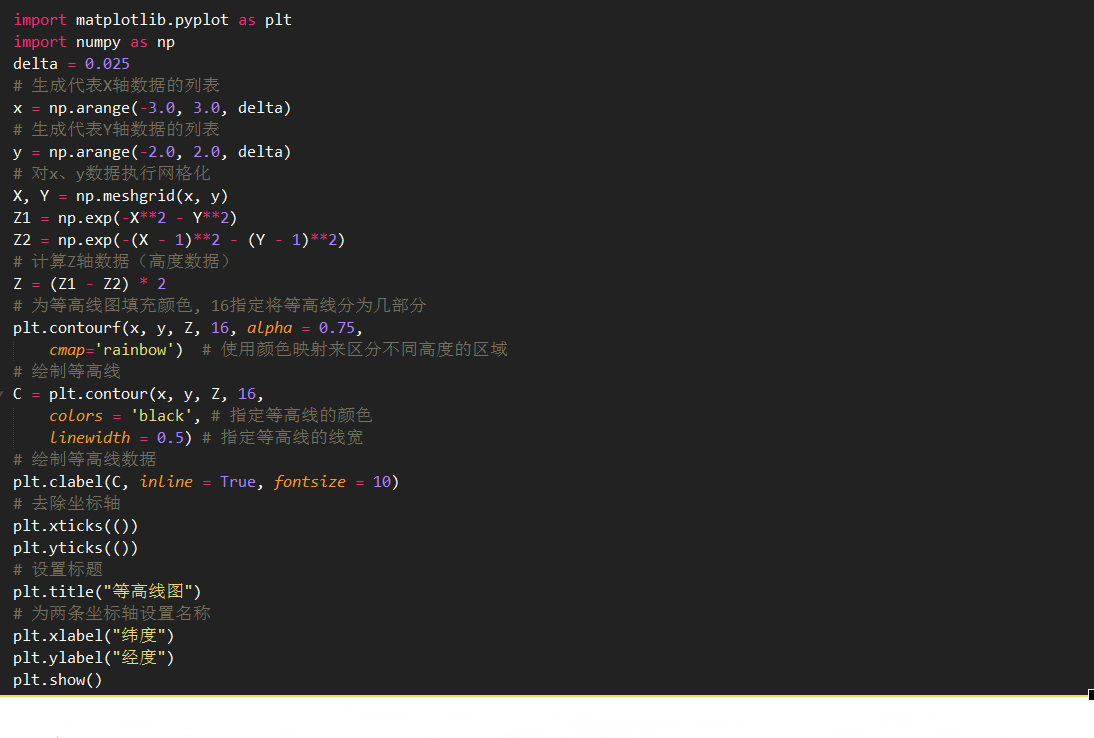上面程序中，第 16 行代码用于为等高线图填充颜色，此处指定了 cmap 参数，这意味着程序将会使用不同的颜色映射来区分不同高度的区域。

程序中第 19 行代码调用 contour() 函数来绘制等高线。运行上面程序，可以看到如图 1 所示的效果。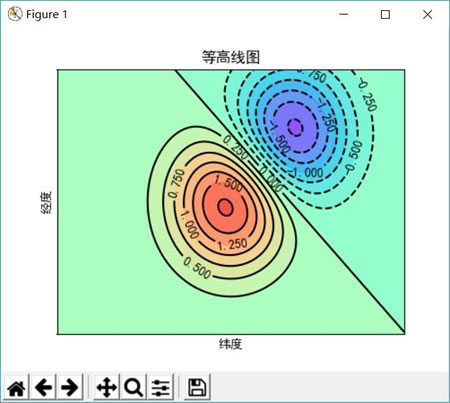图 1 contour和contourf绘制等高线图

更多相关内容
• # 如题，如何把>0.5的区域填色，而其他区域不填色？  ... names = ['num','lon','lat...con = m.contourf(xi, yi, zi,1,alpha=0.8) #画线的label plt.clabel(con,inline=True,fontsize=10,colors='k') 
• ## matplotlib.pyplot contourf()函数的使用

万次阅读 多人点赞 2018-11-11 19:13:50
matplotlib.pyplot contourf  coutour([X, Y,] Z,[levels], **kwargs) 是来绘制等高线的，contour和...除非另有说明，否则两个版本的函数是相同的。 参数： X,Y：类似数组，可选   为Z的坐标值 ...

matplotlib.pyplot contourf

coutour([X, Y,] Z,[levels], **kwargs)

是来绘制等高线的，contour和contourf都是画三维等高线图的，不同点在于contour() 是绘制轮廓线，contourf()会填充轮廓。除非另有说明，否则两个版本的函数是相同的。

 参数： X,Y：类似数组，可选 为Z中的坐标值 当 X,Y,Z 都是 2 维数组时，它们的形状必须相同。如果都是 1 维数组时，len(X)是 Z 的列数，而 len(Y) 是 Z 中的行数。（例如，经由创建numpy.meshgrid()） Z：类似矩阵 绘制轮廓的高度值 levels：int或类似数组，可选 确定轮廓线/区域的数量和位置 其他参数： aalpha：float ，可选 alpha混合值，介于0（透明）和1（不透明）之间。 cmap：str或colormap ，可选 Colormap用于将数据值（浮点数）从间隔转换为相应Colormap表示的RGBA颜色。用于将数据缩放到间隔中看 。

Example：

要画出等高线，核心函数是plt.contourf()，但在这个函数中输入的参数是x,y对应的网格数据以及此网格对应的高度值，因此我们调用np.meshgrid(x,y)把x,y值转换成网格数据：

import numpy as np
import pandas as pd
import matplotlib.pyplot as plt

# 计算x,y坐标对应的高度值
def f(x, y):
return (1-x/2+x**3+y**5) * np.exp(-x**2-y**2)

# 生成x,y的数据
n = 256
x = np.linspace(-3, 3, n)
y = np.linspace(-3, 3, n)

# 把x,y数据生成mesh网格状的数据，因为等高线的显示是在网格的基础上添加上高度值
X, Y = np.meshgrid(x, y)

# 填充等高线
plt.contourf(X, Y, f(X, Y))
# 显示图表
plt.show()


显示的图为：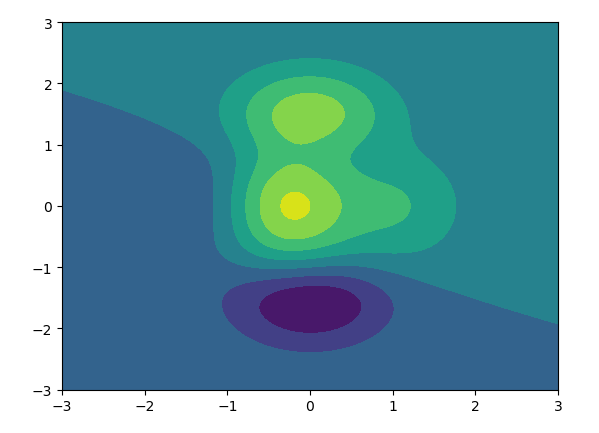如果想显示热力图，那只要在plt.contourf()函数中添加属性cmap=plt.cm.hot就能显示热力图，其中cmap代表为color map，我们把color map映射成hot(热力图)

# 填充等高线
plt.contourf(X, Y, f(X, Y), cmap=plt.cm.hot)

显示的图为：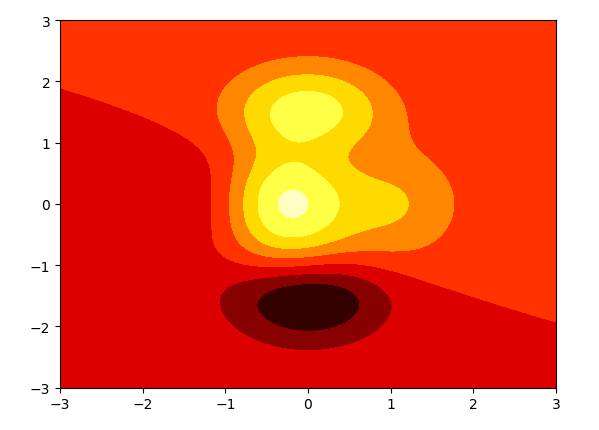参考文章：

用matplotlib画等高线图详解

展开全文matplotlib
• 我一直在使用from_levels_and_colors函数,因此我可以在pcolormesh图上使用扩展颜色条,类似于contourf.这是我的示例contourf plot：import numpy as npimport matplotlib.pyplot as plta = np.arange(12)[:,np....

我一直在使用from_levels_and_colors函数,因此我可以在pcolormesh图上使用扩展颜色条,类似于contourf.这是我的示例contourf plot：

import numpy as np

import matplotlib.pyplot as plt

a = np.arange(12)[:,np.newaxis] * np.ones(8)

levels = np.arange(1.5, 10, 2)

plt.contourf(a, cmap='RdYlBu', levels=levels, extend='both')

plt.colorbar()为了产生类似的pcolormesh图,我需要提供一系列颜色,所以我有：

from matplotlib.colors import from_levels_and_colors

n_colors = len(levels) + 1

cmap = plt.get_cmap('RdYlBu', n_colors)

colors = cmap(range(cmap.N))

cmap, norm = from_levels_and_colors(levels, colors, extend='both')

plt.pcolormesh(a, cmap=cmap, norm=norm)

plt.colorbar()pcolormesh中的中间四种颜色比contourf中的颜色浅.我如何选择它们才能匹配？

解决方法:

问题是,contourf图的颜色是从相应间隔的中间获取的.要为pcolor绘图复制相同的行为,您需要选择颜色不仅仅是与colormap(colors = cmap(range(cmap.N)))的间距相等,而是作为地图的两个端点和相应的意味着水平边界之间.

cnorm = plt.Normalize(vmin=levels,vmax=levels[-1])

clevels = [levels] + list(0.5*(levels[1:]+levels[:-1])) + [levels[-1]]

colors=plt.cm.RdYlBu(cnorm(clevels))

完整代码：

import numpy as np

import matplotlib.pyplot as plt

import matplotlib.colors

a = np.arange(12)[:,np.newaxis] * np.ones(8)

levels = np.arange(1.5, 10, 2)

fig, (ax,ax2) = plt.subplots(ncols=2)

ax.set_title("contourf")

cf = ax.contourf(a, levels=levels, cmap='RdYlBu', extend='both') #,

fig.colorbar(cf, ax=ax)

##### pcolormesh

cnorm = plt.Normalize(vmin=levels,vmax=levels[-1])

clevels = [levels] + list(0.5*(levels[1:]+levels[:-1])) + [levels[-1]]

colors=plt.cm.RdYlBu(cnorm(clevels))

cmap, norm = matplotlib.colors.from_levels_and_colors(levels, colors, extend='both')

cf = ax2.pcolormesh(a, cmap=cmap, norm=norm)

ax2.set_title("pcolormesh")

fig.colorbar(cf,ax=ax2)

plt.tight_layout()

plt.show()为了更好地理解解决方案,您可能希望替换行cmap,norm = matplotlib.colors.from_levels_and_colors(levels,colors,extend =’both’)

norm=matplotlib.colors.BoundaryNorm(levels, ncolors=len(levels)-1)

cmap = matplotlib.colors.ListedColormap(colors[1:-1], N=len(levels)-1)

cmap.set_under(colors)

cmap.set_over(colors[-1])

cmap.colorbar_extend = "both"

这可以使其更清晰,最终使用的颜色和色彩图源自何处.

标签：python,matplotlib

展开全文• 使用极坐标画等直线图，可以用极地图的ax.contour或ax.contourf 。 1.变量计算 每个象限的风速，距离就不再说怎么画了，这里说下角度的计算。 两个经纬度之间的夹角（与正北方向的夹角）： # 点1到点2方向沿逆时针...

任务：将每个象限的风速，距离，角度绘制成极坐标轮廓图。
使用极坐标画等直线图，可以用极地图的ax.contourax.contourf

### 1.变量计算

每个象限的风速，距离就不再说怎么画了，这里说下角度的计算。

两个经纬度之间的夹角（与正北方向的夹角）：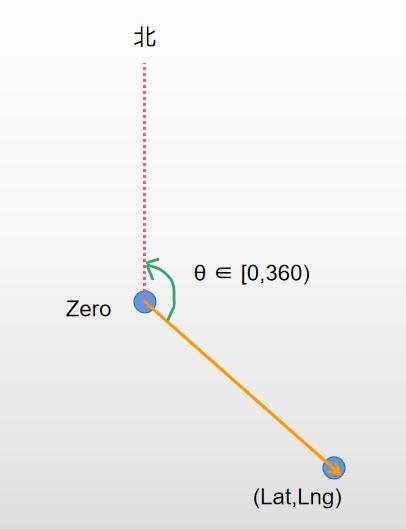# 点1到点2方向沿逆时针方向转到正北方向的夹角
def LatLng2Degree(LatZero,LngZero,Lat,Lng):
"""
Args:
point p1(latA, lonA)
point p2(latB, lonB)
Returns:
bearing between the two GPS points,
default: the basis of heading direction is north
"""
brng = math.degrees(math.atan2(y, x))
brng = (brng + 360) % 360
return brng


四个象限的距离，风速，角度一一对应。

### 2. 极坐标绘制ax.contourf

import numpy as np
import matplotlib.pyplot as plt

#-- Generate Data -----------------------------------------
# Using linspace so that the endpoint of 360 is included...
zeniths = np.arange(0, 70, 10)

r, theta = np.meshgrid(zeniths, azimuths)
values = np.random.random((azimuths.size, zeniths.size))

#-- Plot... ------------------------------------------------
fig, ax = plt.subplots(subplot_kw=dict(projection='polar'))
ax.contourf(theta, r, values)

plt.show()


首先，创建极坐标轴，绘制轮廓图。

fig, ax = subplots(subplot_kw=dict(projection='polar'))
cax = ax.contourf(theta, r, values, nlevels)


参数分别为theta:角度，r:半径，values:轮廓的实际值，nlevels：要绘制的轮廓图的层数。

这里theta， r， values 都需要是二维数组，需要通过列表转化为列。相当于需要先构造网格矩阵，对于坐标轴，一般使用

r, theta = np.meshgrid(zeniths, azimuths)


np.meshgrid函数去构造。

一般情况下，theta， r， values的对应关系是：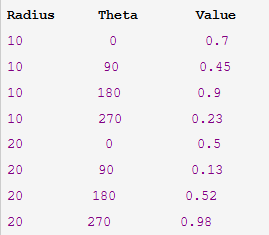可以通过reshape将值转化为二维数组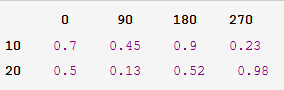这里可以使用：

theta = np.radians(azimuths)
zeniths = np.array(zeniths)

values = np.array(values)
values = values.reshape(len(azimuths), len(zeniths))



但当theta， r， values一一对应时，需要插值实现。这里记得将角度转化为弧度

r = np.array(r)
v = np.array(v)
angle = np.array(angle)
angle1, r1 = np.meshgrid(angle, r)  # r和angle构造网格矩阵
v_new = griddata((angle, r), v, (angle1, r1), method='linear')
cax = ax.contourf(angle1, r1, v_new, 20, cmap='jet')


这里python插值可以参考：python插值（scipy.interpolate模块的griddata和Rbf）

可以解释一下使用Rbf插值会报错的原因，是因为有的方位角相同，比如多行是0度，这样在使用Rbf插值的时候，要求矩阵可逆，则会出现报错的情况。

插值完以后，这里还有一个点，就是把0度调整为正北方向。

ax.set_theta_zero_location("N")
ax.set_theta_direction(-1)


运行结果：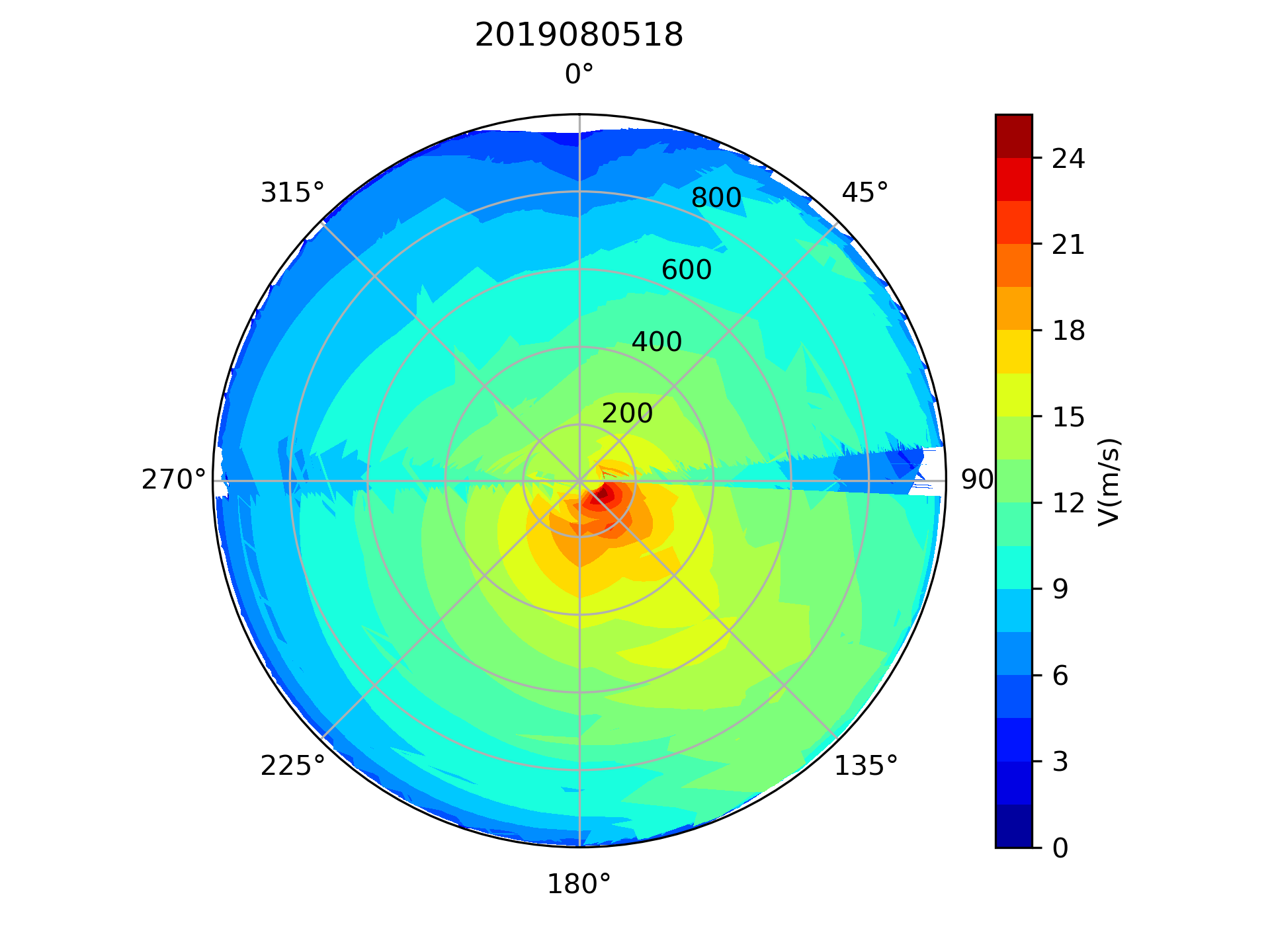可以看到四个象限的风速就用极坐标轮廓图描述出来了。

展开全文• 先讲解plt.contourf函数，然后用plt.contourf绘制决策边界 contourf contourf(*args, data=None, **kwargs) Plot contours. Call signature:: contour([X, Y,] Z, [levels], **kwargs) .contour and ....决策边界 机器学习
• python Basemap 绘制contourf 图 Basemap 为python 附加的地图包，当需要绘制地图时通过Basemap 可以方便地调用，绘制地图边界等。 导入数据 参考博客python HDF 文件读取 的数据Lat Lon 和data 绘图 导入...
• 摘要这一篇文章主要介绍如何绘制轮廓线和对轮廓进行填充, 也就是函数contourf的简单使用.... 其实就是主要介绍contourf函数的简单使用方式. 主要会分为三个方面进行说明, 首先是函数中参数level...
• numpy的meshgrid函数 np.c_和np.r_的用法解析 Contours 等高线图 关于plt.cm.Spectral 1. np.meshgrid(x,y) 与 np.c_[ ] xx, yy = np.meshgrid(x,y)的作用是在二维平面中将每一个x和每一个y分别对应起来，编织成...
• ## Python绘图实用函数

千次阅读 2020-11-30 04:17:31
import pylab as plpl.plot(0,0,‘ro’)pl.show()# pl.savefig(‘fig.jpg’)pylab很多函数和matlab一样，参数的含义也是相似的。例子plot()函数在原点处画了一个红色的圆点。pylab在画图时的完整过程应该是先...
• 先设置一下图像跳出显示：File | Settings | Tools | Python Scientific | Show plots in toolwindow 0. 画散点图 机器学习同时画多个类别的散点图 # 绘制散点图,s是点的面积，marker是点的形状，alpha是点的透明度 ...
• 函数优化涉及从目标函数中找到产生最佳值的输入。优化算法在输入变量的搜索空间中导航以定位最佳位置，目标函数的形状和算法在搜索空间中的行为在现实世界中都是不透明的。因此，通常使用简单的低维函数来研究...
• import numpy as np import matplotlib.pyplot as plt x, y = np.mgrid[-2:2:500j, -2:2:500j] z = (x ** 2 + y ** 2 - 1) ** 3 -...plt.contourf(x, y, z, levels=[-1, 0]) plt.gca().set_aspect('equal') plt.show()
• contour做的云图不是填充的，而contourf画的云图是填充的来两个例子一目了然，代码可用。(本文例子来自matplotlib管网)contour函数contour([X, Y,] Z, [levels], **kwargs)X, Y， The coordinates of the values in ...
• 多元函数的本质是一种关系，是两个集合间一种确定的对应关系。多元函数是后续人工智能的基础，先可视化呈现，后续再学习一下求导。设D为一个非空的n 元有序数组的集合， f为某一确定的对应规则。若...数据可视化 graphviz qt opencv
• 我在“x-cord”、“y-cord”、...我放弃了在pgfplots和matlab实现这一点的尝试，并想试试python。用这些脚本的任何一个都可以。python脚本如下import numpy as npfrom scipy.interpolate import griddataimport...
• plt.contour是python中用于画等高线的函数，这里介绍一下plt.contour的使用。 文章目录使用示例plt.contour()函数本身plt.contour()图的坐标叮！ 使用示例 import numpy as np import matplotlib.pyplot as plt ...
• 在做吴恩达老师的深度学习课程作业时，发现决策边界...绘制梯度下降算法图形或是决策边界，核心便在于知道plt.contourf函数的用法 plt.contourf函数 这里参考https://blog.csdn.net/qq_44669578/article/details/103机器学习 深度学习
• 我用了两个色条在一个紧凑布局的图形显示两个图形。因此，一个垂直的色条似乎太长了，我想调整它的大小。所以，我编码如下：CS1 = plt.contourf(X1,Y1,Z1,15,cmap=plt.cm.Greys,vmax=abs(Z1).max(), vmin=abs(Z1)....
• import pylab as plpl.plot(0,0,‘ro’)pl.show()# pl.savefig(‘fig.jpg’)pylab很多函数和matlab一样，参数的含义也是相似的。例子plot()函数在原点处画了一个红色的圆点。pylab在画图时的完整过程应该是先...
• sharey=ax) bx.contourf(X, Y, Z, crange) bx.set_title('Spline') bx.text(0.05, 0.98, 'b)', ha='left', va='top', transform=bx.transAxes, bbox=bbox) cx = fig.add_subplot(1, 3, 3, sharex=ax, sharey=ax) cx...
• 连续小波变换可用的小波： **A wide range of continous wavelets are now available. These include the following:** Gaussian wavelets (gaus1…gaus8`) Mexican hat wavelet (mexh) Morlet wavelet ...开发语言 后端
• ## Python 绘图各种函数

千次阅读 2020-01-11 15:56:00
python下一般使用matplotlib包下的pyplot，所以在import的时候： 一般import matplotlib.pyplot as plt方便使用它的绘图函数。 通用函数 show() 显示绘图窗口，把画的图显示在窗口。 subplot() 用于添加子图像，...
• 来自 莫烦python的总结。 打印图像 imshow a = np.array([0.313,0.365,0.423, 0.365,0.439,0.525, 0.423,0.525,0.651]).reshape(3,3) plt.imshow(a,interpolation='nearest',cmap='bone',origin='lower') # origin...numpy
• viridis, viridis_r, winter, winter_r').split(', ') for i in mm: plt.figure() plt.contourf(tfr.data[6,2,:,:],cmap=i) fig = plt.gcf() fig.savefig(r'./figure/' + i + '.png') plt.close() 得到结果如下：
• 所以我可以在这个帧绘制一个直方图图，但是当我使用kde生成数据并使用fill覆盖到{}时，它不会旋转。在import pylab as pltimport seaborn as snsfrom scipy.stats import gaussian_kdeimport numpy as npfrom ...
• 在多个图进行比较时，用同样的颜色区间对比更明显一些，但是我找了很久，问了不少人，一直没有解决这个问题，后来随便乱写了一下，发现contourf第4个参数用数组有奇效import numpy as np import matplotlib.pyplot ...matplotlib
• plt.figure() ax = fig.add_subplot(111) cores = ax.contourf(surface[:], vmin=0, vmax=40) cbar = plt.colorbar(cores) 我也试过这个： cbar = plt.colorbar(cores, extend='both', extendrect=True) cbar.set_...
• 测试函数主要是用来评估优化算法特性的，这里我用python3绘制了部分测试函数的图像。具体的测试函数可以结合维基百科来了解。想要显示某个测试函数的图片把代码结尾对应的注释去掉即可，具体代码如下：import numpy ......